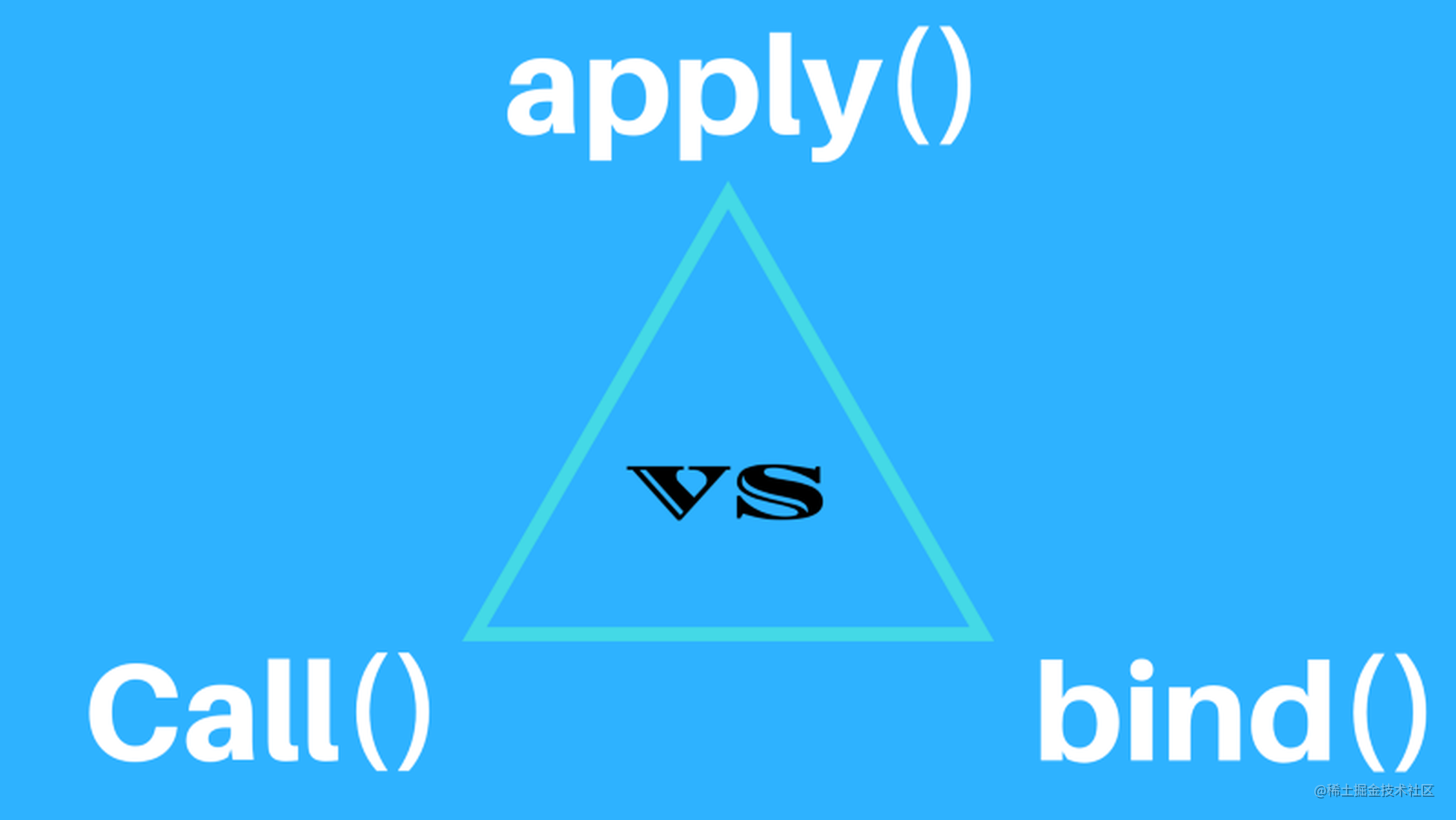# call,apply,bind 的使用与区别

## call

call() 方法是使用一个指定的`this`值和单独给出的一个或多个参数来调用一个函数。

• function.call(thisArg, arg1, arg2, ...)。
• thisArg：可选的。在 function 函数运行时使用的 this 值。
• arg1, arg2, ...：指定的参数列表

## apply

apply() 方法调用一个具有给定 `this` 值的函数，以及以一个数组（或类数组对象）的形式提供的参数。

• function.apply(thisArg, argsArray)
• thisArg：必选的。在 function 函数运行时使用的 this 值
• argsArray：可选的。一个数组或者类数组对象，其中的数组元素将作为单独的参数传给 func 函数。

## bind

bind() 方法创建一个新的函数，在 bind() 被调用时，这个新函数的 `this` 被指定为 bind() 的第一个参数，而其余参数将作为新函数的参数，供调用时使用。

• function.bind(thisArg, arg1, arg2, ...)
• thisArg：调用绑定函数时作为 this 参数传递给目标函数的值。
• arg1, arg2, ...：当目标函数被调用时，被预置入绑定函数的参数列表中的参数。

## call,apply,bind 区别

• call：可以改变函数指向，第一个参数是要改变指向的对象(this指向第一个参数)，之后的参数形式是 arg1, arg2... 的形式。

• apply：基本同 call(this指向第一个参数)，不同点在于第二个参数是一个数组 [arg1, arg2...]。

• bind：改变 this 作用域会返回一个新的函数，这个函数不会马上执行。

# 手写实现call

``````function myCall(context = window, ...args) {
const _context = Object(context)

// 改变this的指向
_context.fn = this;

// 调用这个方法，将剩余参数传递进去
_context.fn(...args);

// 将这个方法的执行结果传给 result
let result = _context.fn();

// 删除这个变量
delete _context.fn;

return result;
}

Function.prototype.myCall = myCall;

// test

this.a=1;
const fn = function () {
this.a = 2;
console.log(this.a);
};

fn.myCall(fn);

# 手写实现apply

``````Function.prototype.myApply = function (context = window, args = []) {
const _context = Object(context)

_context.fn = this; // this是调用call的函数

const result = _context.fn(...args);
delete _context.fn
return result;
}

// test

this.a = 1;

const fn = function() {
this.a = 2;
console.log(this.a);
}

fn.myApply(fn);

``````Function.prototype.myBind = function(context, ...args) {
const _this = this;
return function Bind(...newArgs) {
// 如果是通过 new 调用的，绑定 this 为实例对象
if (this instanceof Bind) {
return _this.myApply(this, [...args, ...newArgs]);
}
// 普通函数
return _this.myApply(context, [...args, ...newArgs]);
};
}

// 第二种方法
Function.prototype.myBind = function (context, ...args) {
const _context = Object(context);
return (...newArgs) => {
return this.myApply(_context, [...args, ...newArgs]);
};
};

//test

let b = {
num: 1,
};
function f1() {
console.log('====', this.num);
}

const f2 = f1.myBind(b);
f2();